# Air Density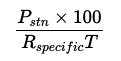Pstn = station pressure in millibars (mb)

T = temperature in Kelvin

Rspeciic = specific gas constant for dry air (287.058 J/(kg·K))

# Delta T

Delta T, ΔT, is used in agriculture to indicate acceptable conditions for spraying pesticides and fertilizers. It is simply the difference between the air temperature (aka "dry bulb temperature") and the wet bulb temperature:

ΔT = TTwb

# Dew Point Temperature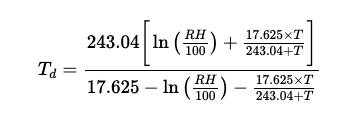Td = dew point in degrees Celsius (°C)

T = temperature in degrees Celsius (°C)

RH = relative humidity (%)

# Feels Like Temperature

The Feels Like temperature is equal to the Heat Index if the temperature is at or above 80°F and the relative humidity is at or above 40%. Alternatively, the Feels Like temperature is equal to Wind Chill if the temperature is at or below 50°F and wind speeds are above 3mph. If neither condition applies, the Feels Like temperature is equal to the air temperature.

a b c d

# Heat Index Temperature

Source: Weather.gov

Heat Index is calculated for temperatures at or above 80°F and a relative humidity at or above 40%.

Thi = −42.379 + (2.04901523 × T) + (10.1433127 × RH) − (0.22475541 × T × RH) − (6.83783 × 10−3 × T2) − (5.481717 × 10−2 × RH2) + (1.22874 × 10−3 × T2 × RH) + (8.5282 × 10−4 × T × RH2) − (1.99 × 10−6 × T2 × RH2)

T = temperature in degrees Fahrenheit (°F)

RH = relative humidity (%)

# Pressure Trend

The Pressure Trend description is determined by the rate of change over the past 3 hours.

ΔP = P0hP3h

P0h = the latest pressure reading in millibars (mb)

P3h = pressure reading 3 hours ago in millibars (mb)

DescriptionRate
Steady−1 mb < ΔP < 1mb
FallingΔP ≤ -1mb
RisingΔP ≥ 1mb

# Rain Rate

The Rain Rate description is set according to the latest one minute accumulation, extrapolated to an hourly rate.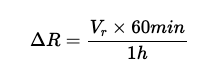Vr = rain accumulation in millimeters over one minute (mm/min)

DescriptionRate
NoneΔR = 0mm/h
Very Light0mm/h < ΔR < 0.25mm/h
Light0.25mm/h ≤ ΔR < 1.0mm/h
Moderate1.0mm/h ≤ ΔR < 4.0mm/h
Heavy4.0mm/h ≤ ΔR < 16.0mm/h
Very Heavy16.0mm/h ≤ ΔR < 50.0mm/h
ExtremeΔR ≥ 50.0mm/h

# Sea Level Pressure

Source: AMSPsta = station pressure in millibars (mb)

P0 = standard sea level pressure (1013.25mb)

Rd = gas constant for dry air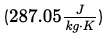γs = standard atmosphere lapse rate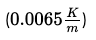g = gravity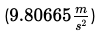hel = ground elevation in meters (m)

hag = station height above ground in meters (m)

T0 = standard sea level temperature (288.15K)

# Vapor Pressure

Source: Weather.gov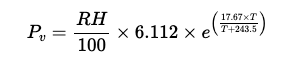Vapor pressure, Pv can be estimated in units of millibar (mb) as follows:

T = temperature in degrees Celsius (°C)

RH = relative humidity (%)

# Wet Bulb Temperature

Source: Weather.gov

Wet Bulb Temperature (Twb), is determined using the following formulas for actual vapor pressure (Pv) and the vapor pressure related to wet bulb temperature (Pv,wb) in millibar (mb):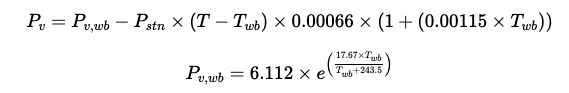T = temperature in degrees Fahrenheit (°F)

V = wind speed in mph

# Wind Chill Temperature

Source: Weather.gov

Wind Chill is calculated for temperatures at or below 50°F and wind speeds above 3mph.

Twc = 35.74 + (0.6215 × T) − (35.75 × V0.16) + (0.4275 × T × V0.16)

T = temperature in degrees Fahrenheit (°F)

V = wind speed in mph